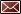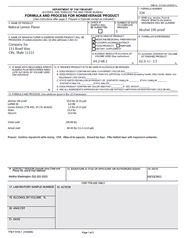Get Email Updates Back to Nonbeverage Products Laboratory page

# Drawback Tutorial

Example formulas

Washed extract (with multiple sources of ethanol)

Calculating items 9 and 10 for this extract is more complex than the previous examples.Step 1. Item 9 calculation – Locate the eligible ethanol in the formula.  The only source is the 190 proof ethanol (pounds of ethanol are converted to gallons as in the previous examples).  Note that the Lemon Extract has a TTB approval for drawback.  first, multiply the volume of 190 proof ethanol (8.10 gallons) by the % ethanol 8.10 gallons × 0.95 = 7.70 gallons of absolute ethanol Next, divide by the final yield low end of yield range 7.70 gallons of absolute ethanol ÷ 11.3 gallons = 0.680 or 68.1% high end of yield range 7.70 gallons of absolute ethanol ÷ 12.0 gallons = 0.642 or 64.2% This provides the range for the eligible alcohol in item 9. Click on the image to enlarge

Step 2. Item 10 calculation - For this, a determination of the concentration of the ethanol in the starting menstruum must be made (ethanol and water along with other dissolved components (not the oil!)).

 Volume of Starting menstruum = sum of all soluble components Ethanol = 55.0 pounds ÷ 6.794 pounds/gallon = 8.10 gallons Lemon Extract = 0.14 gallons Glycerin = 23.0 pounds ÷ 9.79 pounds/gallon = 2.35 gallons Water = 15.0 pounds ÷ 8.328 pounds/gallon = 1.80 gallons ___________________________________________                Sum = 12.39 gallons of starting menstruum

Use the sum (12.39 gallons) in the denominator of the following equation:

Item 10 = [gallons of absolute ethanol] ÷ [volume (in gallons) of starting menstruum]

Where gallons of absolute ethanol = 190 proof ethanol + ethanol from TTB # 8180

 8.10 gal × 0.95 = 7.70 gallons of absolute ethanol 0.14 gal × 0.671 = 0.09 gallons of absolute ethanol ___________________________________________ Sum = 7.79 gallons of absolute ethanol

Divide this number by the volume of the starting menstruum to get item 10 7.79 gallons of absolute ethanol ÷ 12.39 gallons = 0.629 or 62.9%

For extracts, the tolerance used for filtered products is applied to item 10 (see the tolerance table).